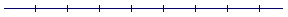Is a line really composed of points?An Approach

t o

C A L C U L U S

Appendix 2

# IS A LINE REALLY COMPOSED OF POINTS?

THE WHOLE PROBLEM of a continuum of numbers began with the assumption, the concept, that a line—the x-axis—is composed of points, and that to every point there must correspond a number. But does calculus really require that? And is a line really composed of points?

Let us begin by remembering that points and lines are ideas. A line is the idea of length only. A point is the idea of position only. As ideas, points and lines exist. Is that enough?

Euclid postulated that a straight line will exist logically—when it has been drawn. We will have brought a representation of that idea into this world. As for a "point," it is not a thing. It is the word we use for the boundary of a line or of an interval. Hence a point will exist when we have drawn a straight line, or when we indicate a boundary. "Let x take values in the open interval between −1 and 5." A point will exist when we name the value of a variable. "Let x have the value 2." For, 2 is the coördinate of the endpoint of a distance from 0.

(We may say there are an infinite number of points on a line, which is a brief way of saying that there is no limit to the number of points we could indicate.)

Points exist potentially. Calculus has no need for anything more. Points are like pitches on a guitar string. A pitch does not exist until it is sounded: a guitar string is not composed of pitches. And the x-axis is not composed of positions.

(To accept that an infinite number of points of zero magnitude will add up to a positive magnitude, calls for credulity more typical of the demands of religion. What is more, it approves division by 0.)

One could of course completely redefine the meaning of the word composed and the word point.

"When I use a word," Humpty Dumpty said, in rather a scornful tone, "it means just what I choose it to mean—neither more nor less."

"The question is," said Alice, "whether you can make words mean so many different things."

"The question is," said Humpty Dumpty, "which is to be master—that's all."

Alice in Wonderland

Say, however, that a line were composed of points. Now the most common and important application of calculus is to motion, where the independent variable is time t. Then if the abstract x-axis is composed of points, its application to time must also be composed of points. That is, the t-axis will be composed of points, or, we would say, instants. Is that a valid presumption?

First, like any continuous quantity, time is not inherently composed of intervals; yet we can conveniently decompose time into any intervals,any units of measure—hours, minutes, seconds—we please, however small.

Time will then be composed of those intervals, which will have common boundaries, to which we give the name "instants."

But time cannot be composed of instants—they are not a unit of time.

If time did consist solely of instants, then at any one instant a body is at rest. It cannot move to another instant, because no time elapses. In other words, there could be no motion. That is the arrow paradox of Zeno.  But because time continues and has no inherent components—it is not composed of instants—that paradox is not valid.

Since the t-axis then is not composed of points, then neither can the x-axis—the so-called real line—of which time is but an application.

Thus a continuum of numbers not only does not exist, it is not necessary. If a function is continuous, then it will be continuous at every value of x we name.Appendix 1:  Are the real numbers really numbers?